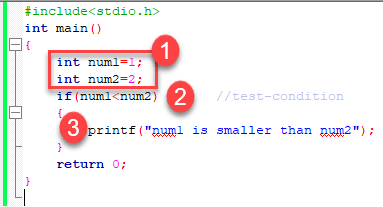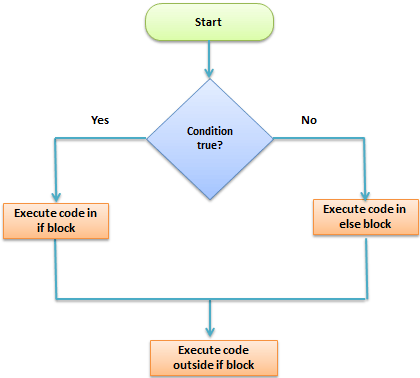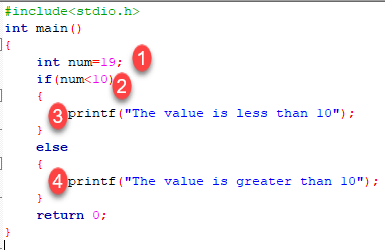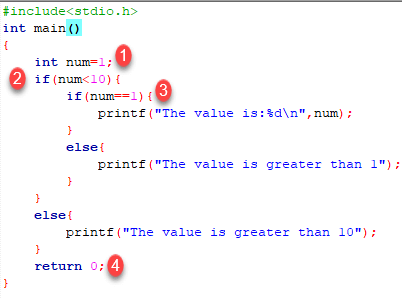# C Conditional Statement: IF, IF Else and Nested IF Else with Example

## What is a Conditional Statement?

In a ‘C’ program are executed sequentially. This happens when there is no condition around the statements. If you put some condition for a block of statements the flow of execution might change based on the result evaluated by the condition. This process is referred to as decision making in ‘C.’ The decision-making statements are also called as control statements.

In ‘C’ programming conditional statements are possible with the help of the following two constructs:

1. If statement

2. If-else statement

It is also called as branching as a program decides which statement to execute based on the result of the evaluated condition.

In this tutorial, you will learn-

## If statement

It is one of the powerful conditional statement. If statement is responsible for modifying the flow of execution of a program. If statement is always used with a condition. The condition is evaluated first before executing any statement inside the body of If. The syntax for if statement is as follows:

``` if (condition)
instruction; ```

The condition evaluates to either true or false. True is always a non-zero value, and false is a value that contains zero. Instructions can be a single instruction or a code block enclosed by curly braces { }.

Following program illustrates the use of if construct in ‘C’ programming:

```#include<stdio.h>
int main()
{
int num1=1;
int num2=2;
if(num1<num2)		//test-condition
{
printf("num1 is smaller than num2");
}
return 0;
}
```

Output:

```num1 is smaller than num2
```

The above program illustrates the use of if construct to check equality of two numbers.1. In the above program, we have initialized two variables with num1, num2 with value as 1, 2 respectively.
2. Then, we have used if with a test-expression to check which number is the smallest and which number is the largest. We have used a relational expression in if construct. Since the value of num1 is smaller than num2, the condition will evaluate to true.
3. Thus it will print the statement inside the block of If. After that, the control will go outside of the block and program will be terminated with a successful result.

## Relational Operators

C has six relational operators that can be used to formulate a Boolean expression for making a decision and testing conditions, which returns true or false :

< less than

<= less than or equal to

> greater than

>= greater than or equal to

== equal to

!= not equal to

Notice that the equal test (==) is different from the assignment operator (=) because it is one of the most common problems that a programmer faces by mixing them up.

For example:

```int x = 41;
x =x+ 1;
if (x == 42) {
printf("You succeed!");}
```

Output :

` You succeed `

Keep in mind that a condition that evaluates to a non-zero value is considered as true.

For example:

```int present = 1;
if (present)
printf("There is someone present in the classroom \n");
```

Output :

`There is someone present in the classroom `

## The If-Else statementThe if-else is statement is an extended version of If. The general form of if-else is as follows:

```if (test-expression)
{
True block of statements
}
Else
{
False block of statements
}
Statements;
```

n this type of a construct, if the value of test-expression is true, then the true block of statements will be executed. If the value of test-expression if false, then the false block of statements will be executed. In any case, after the execution, the control will be automatically transferred to the statements appearing outside the block of If.

Following programs illustrate the use of the if-else construct:

We will initialize a variable with some value and write a program to determine if the value is less than ten or greater than ten.

Let’s start.

```#include<stdio.h>
int main()
{
int num=19;
if(num<10)
{
printf("The value is less than 10");
}
else
{
printf("The value is greater than 10");
}
return 0;
}
```

Output:

```The value is greater than 10
```1. We have initialized a variable with value 19. We have to find out whether the number is bigger or smaller than 10 using a ‘C’ program. To do this, we have used the if-else construct.
2. Here we have provided a condition num<10 because we have to compare our value with 10.
3. As you can see the first block is always a true block which means, if the value of test-expression is true then the first block which is If, will be executed.
4. The second block is an else block. This block contains the statements which will be executed if the value of the test-expression becomes false. In our program, the value of num is greater than ten hence the test-condition becomes false and else block is executed. Thus, our output will be from an else block which is “The value is greater than 10”. After the if-else, the program will terminate with a successful result.

In ‘C’ programming we can use multiple if-else constructs within each other which are referred to as nesting of if-else statements.

## Conditional Expressions

There is another way to express an if-else statement is by introducing the ?: operator. In a conditional expression the ?: operator has only one statement associated with the if and the else.

For example:

```#include <stdio.h>
int main() {
int y;
int x = 2;
y = (x >= 6) ?  6 : x;/* This is equivalent to:  if (x >= 5)    y = 5;  else    y = x; */
printf("y =%d ",y);
return 0;}```

Output :

`y =2`

## Nested If-else Statements

When a series of decision is required, nested if-else is used. Nesting means using one if-else construct within another one.

Let’s write a program to illustrate the use of nested if-else.

```#include<stdio.h>
int main()
{
int num=1;
if(num<10)
{
if(num==1)
{
printf("The value is:%d\n",num);
}
else
{
printf("The value is greater than 1");
}
}
else
{
printf("The value is greater than 10");
}
return 0;
}
```

Output:

```The value is:1
```

The above program checks if a number is less or greater than 10 and prints the result using nested if-else construct.1. Firstly, we have declared a variable num with value as 1. Then we have used if-else construct.
2. In the outer if-else, the condition provided checks if a number is less than 10. If the condition is true then and only then it will execute the inner loop. In this case, the condition is true hence the inner block is processed.
3. In the inner block, we again have a condition that checks if our variable contains the value 1 or not. When a condition is true, then it will process the If block otherwise it will process an else block. In this case, the condition is true hence the If a block is executed and the value is printed on the output screen.
4. The above program will print the value of a variable and exit with success.

Try changing the value of variable see how the program behaves.

NOTE: In nested if-else, we have to be careful with the indentation because multiple if-else constructs are involved in this process, so it becomes difficult to figure out individual constructs. Proper indentation makes it easy to read the program.

## Nested Else-if statements

Nested else-if is used when multipath decisions are required.

The general syntax of how else-if ladders are constructed in ‘C’ programming is as follows:

``` if (test - expression 1) {
statement1;
} else if (test - expression 2) {
Statement2;
} else if (test - expression 3) {
Statement3;
} else if (test - expression n) {
Statement n;
} else {
default;
}
Statement x;
```

This type of structure is known as the else-if ladder. This chain generally looks like a ladder hence it is also called as an else-if ladder. The test-expressions are evaluated from top to bottom. Whenever a true test-expression if found, statement associated with it is executed. When all the n test-expressions becomes false, then the default else statement is executed.

Let us see the actual working with the help of a program.

```#include<stdio.h>
int main()
{
int marks=83;
if(marks>75){
printf("First class");
}
else if(marks>65){
printf("Second class");
}
else if(marks>55){
printf("Third class");
}
else{
printf("Fourth class");
}
return 0;
}```

Output:

`First class`

The above program prints the grade as per the marks scored in a test. We have used the else-if ladder construct in the above program.1. We have initialized a variable with marks. In the else-if ladder structure, we have provided various conditions.
2. The value from the variable marks will be compared with the first condition since it is true the statement associated with it will be printed on the output screen.
3. If the first test condition turns out false, then it is compared with the second condition.
4. This process will go on until the all expression is evaluated otherwise control will go out of the else-if ladder, and default statement will be printed.

Try modifying the value and notice the change in the output.

Summary

• Decision making or branching statements are used to select one path based on the result of the evaluated expression.
• It is also called as control statements because it controls the flow of execution of a program.
• ‘C’ provides if, if-else constructs for decision-making statements.
• We can also nest if-else within one another when multiple paths have to be tested.
• The else-if ladder is used when we have to check various ways based upon the result of the expression.

#### Top Courses